July 21

# Runge-Kutta method in MATLAB

lang: en_US

Welcome to our blog on the Runge-Kutta method in MATLAB! If you're searching for an efficient and accurate numerical technique to solve ordinary differential equations (ODEs), you've come to the right place. In this blog post, we will explore the power of the Runge-Kutta method, particularly focusing on its implementation in MATLAB. By the end of this article, you'll have a clear understanding of how to apply the fourth-order Runge-Kutta (RK4) method in MATLAB to obtain precise solutions for ODEs.

We will learn to program a Runge-Kutta 4th-order algorithm in MATLAB to solve ordinary differential equations. Also, we will gain more insights into the MATLAB programming used to define the ODE as a custom function, calculate the intermediate steps using a for loop, and plot the results on a graph.

### What is the 4th-order Runge-Kutta method?

The most commonly used Runge-Kutta method to find the solution of a differential equation is the RK4 method, i.e., the fourth-order Runge-Kutta method. The Runge-Kutta method provides the approximate value of y for a given point x. Only the first-order ODEs can be solved using the Runge Kutta RK4 method.

For the given equation in the form,The formula for Runge-Kutta 4th order method is given as,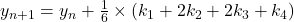whereas,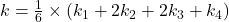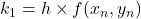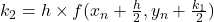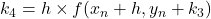## Analytical Method

### Solved example of the Runge-Kutta (RK4) method

Example 1:

Consider an ordinary differential equation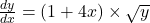, y(0) = 1. Find y(1.5) using the fourth order Runge-Kutta method for given h=0.5 and### Solution:

Given data,       h = 0.5,   x1 = 0,  y1 = 1,  xm = 1.5

We have,So, we can write this as,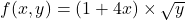To calculate value of k1, k2, k3, k4

Step 1:    for n = 1     i.e, first iteration

k1 = h × f (x1, y1) = 0.5 × f (0, 1) = 0.5 × [(1+4(0)) × 1] = 0.5

k2 = h × f (x1 + h/2, y1 + k1/2) = 0.5 × f (0.25, 1.25) = 0.5 × [(1+4(0.25)) ×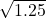] = 1.1180

k3 = h × f (x1 + h/2, y1 + k2/2) = 0.5 × f (0.25, 1.5590) = 1.2485

k4 = h × f (x1 + h, y1 + k3) = 0.5 × f (0.5, 2.2485) = 2.2492

k = (1/6) × (k1 + 2k2 + 2k3 + k4) = (1/6) [0. 5 + 2(1.1180) + 2(1.2485) + 2.2492] = 1.2470

we have,

y2 = y1 + k

y2 = 1 + 1.2470

y2 = 2.2470

Step 2:    for n =2

k1 = h × f (x2, y2) = 0.5 × f (0.5, 2.2470) = 0.5 × [(1+4(0.5)) ×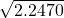] = 2.2484

k2 = h × f (x2 + h/2, y2 + k1/2) = 0.5 × f (0.75, 3.3712) = 3.6721

k3 = h × f (x2 + h/2, y2 + k2/2) = 0.5 × f (0.75, 4.083) = 4.0413

k4 = h × f (x2 + h, y2 + k3) = 0.5 × f (1, 6.2883) = 6.2691

k = (1/6) × (k1 + 2k2 + 2k3 + k4) = 1/6 [2.2484 + 2(3.6721) + 2(4.0413) +6.2691] = 3.9907

we have,

y3 = y2+ k

y3 = 2.2470 + 3.9907

y3 = 6.2377

Step 3:    for n = 3

k1 = h × f (x3, y3) = 0.5 × f (1, 6.2377) = 0.5 × [(1+4(1)) ×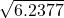] = 6.2438

k2 = h × f (x3 + h/2, y3 + k1/2) = 0.5 × f (1.25, 9.3596) = 9.1780

k3 = h × f (x3 + h/2, y3 + k2/2) = 0.5 × f (1.25, 10.8267) = 9.8711

k4 = h × f (x3 + h, y3 + k3) = 0.5 × f (1.5, 16.1088) = 14.0475

k = (1/6) (k1 + 2k2 + 2k3 + k4) = 1/6 [6.2438 + 2(9.1780) + 2(9.8711) +14.0475] = 9.7315

we have,

y4 = y3+ k

y4 = 6.2377 + 9.7315

y4 = 15.9692

ANS   So, here we can conclude that the value of y at x=1.5 is found to be 15.9692

Therefore,  y(1.5) = 15.9692

## MATLAB Program Method

We have solved the ordinary differential equation analytically using mathematical formulas and procedures, we will now try to solve this problem with the help of MATLAB software. Firstly, we will write the program by taking into account the data given in the problem and using the corresponding mathematical formula.

MATLAB is a high-level programming language that features syntax resembling the English language, making it easier to understand for users.

Step 1:

Here we will declare the standard commands that are used before starting any actual programming. This helps in clearing all the previous data in the command window as well as the workspace.

The (clc) command clears any previous input or output values in the command window.

The (clear) command removes all the variables present in the workspace. You can also use the (clearvars -except<variable name>) command to remove all variables except the one that you declared.

The (format compact) command is used to reduce line spacing in the output displayed in the command window. This makes the output more compact and easier to read.

The (close all) command closes all the previously opened tabs, such as graphs that are generated by earlier program executions.

%% standard commands for clearing any previous data

clc;

clear;

format compact;

close all;

Step 2:

Here in this section, we take the input values from the user for the data already given in the problem. The (input) function is used to assign the user-defined values to a specific variable. All of these values will be given as input to the compiler by the user.

The (d) variable takes the given equation as an input, in the prescribed format.

The (h) is a variable that is assigned to the value of step size. [h = 0.5]

The (a) variable is assigned to the input of the initial value of x from a user. [a = 0]

The (b) variable has the value of x at which the value of y is to be calculated. [b=1.5]

The (c) variable is assigned to the initial value of y. [c = 1]

%% Take user input for step size h and initial values of x and y

d = input('Input the given equation in the format as, @(x,y) equation: ');

h = input('What is the step size h: ');

a = input('Input the initial value of x: ');

b = input('Input the value of x at which y is to be calculated: ');

c = input('Provide the initial value of y: ');

Step 3:

In this section of the code, we will generate a custom function for the given differential equation in the question. Further, we will add (for loop code) to calculate the values of k multiple times. We will also assign a universal argument to the variable x.

The variable x act as a matrix with values between a & b, increasing by h. Consider if the user inputs a=10, b=20 & h=2 values in the command window, then x becomes [10 12 14 16 18 20]

The initial value of y that was taken from the user and assigned to the variable ‘c’ in the previous lines of code, is now assigned to variable y as 1st place value.

The anonymous function that a user will input in the format as @(x,y)  equation, is stored in variable d. Then at this point, the variable d value is assigned to the variable named “func”. This type of inline function is a quick and simple way of creating a function for any calculation. The “@” sign is followed by the inputs (x,y). After some space, a user writes the ordinary differential equation given in the question.

%% Declare the initial values and ODE that is given in the question

x = (a:h:b);         %If range of x is given you can directly put it here.

y(1) = c;

func = d;

%Code for calculating values of K using FOR LOOP

For  i = 1:(length(x)-1)

% In case h is not given calculate by h = x(i+1)-x(i)

k1 = h.*func(x(i),y(i));

k2 = h.*func(x(i)+h/2, y(i)+k1/2);

k3 = h.*func(x(i)+h/2, y(i)+k2/2);

k4 = h.*func(x(i)+h, y(i)+k3);

k = (1/6).*(k1+2*k2+2*k3+k4);

y(i+1) = y(i)+ k;            %Using formula y2=y1+k

end

Then, as we move further in the code, we use a (for loop). The number of times that the for loop will execute is the same as the no. of iterations that we need to perform. Here, the loop will run 3 times as the value of y(1.5) i.e., y4 is calculated using x3. The length of the “x” array is 4 because there are 4 elements in the matrix [0,0.5,1,1.5]. Therefore, the number of loops become (length of x -1), which is 3.

Now we will calculate the values of k1 to k4 using the above syntax. The formula for k1 is used as given in the analytical method, and the values of x(i) and y(i) are assigned as input to the custom function (func). In the first loop, the value of “i” becomes 1, so x(1) = 0 & y(1) = 1 as given in the question. Then by putting these values of x & y in (func) and multiplying it by h, we obtain the value of k1 for 1st iteration as 0.5.

Using the same approach mentioned above, the values of k2, k3 & k4 can be calculated. At last, the value of k is calculated by writing the same formula of k that is used in the analytical method, in the code as it is.

Next when all the values of k1-k4 for 1st iteration are achieved, then by using the last formula y2 = y1+k, we can find “y2” using values of x1 & y1. Here y(i+1) is written because the value of “i” for the first iteration is 1, and we need the value of y2, so y(1+1) = y2.

### IMPORTANT NOTE

The important thing to understand in this section of code is that we have to use (.*) multiplication. In MATLAB this multiplication syntax is used to perform elementwise multiplication, which means multiplying each number individually. This is because, in MATLAB the values assigned to variables are stored in the matrix or array form.

Step 4:

In this step, we will simply store the obtained values of x and y from the loop inside the two new variables X1 & Y1. The double (x) function converts the values in x into double precision. This function is used to convert symbolic numbers into double-precision numbers. This step is simple and often not necessary, but I have used it for simplicity in displaying output & the plot command used in the next step, can take the values of x & y accurately.

%% Collect output values in a matrix, for better understanding and simplicity

X1 = double(x);

Y1 = double(y);

Step 5:

This is the last part of the program where we plot the graph of results obtained from the above calculations. The x and y values up to the 4th point are plotted on the x and y axes respectively. Supportive functions are also used to make the graph look more detailed and attractive.

The plot function is used as per its syntax, first x-axis values (X1) are assigned, and then y-axis values (Y1). Here the (--ob) command specifies the type of graph line to be used. In this case, a dashed line (--) is used, but one can use a solid line (-) or dotted line (:). Furthermore, (o) stands for the points on the graph that are highlighted by the circle. Alternatively, a square symbol or other symbols can be used to plot points on a graph. The (b) stands for the color of the line and circle on the graph.

Next (‘linewidth’,1) function determines the thickness of the line that is drawn by joining points on the graph.

Below are all the functions used to provide more details about the graph. The (title) function gives the heading to the graph, whereas the (xlim & ylim) defines the range of numbers on the two axes. Then the (xlabel & ylabel) functions provide a name or parameter to the x-axis and y-axis. Lastly, the (grid on) command makes the grid lines on the graph visible.

%% Plot graph using the above values of X1 and Y1

plot(X1,Y1,'--ob','linewidth',1)

%Supporting parameters to the graph for better visualization

title('Runge-Kutta method')

xlim([0,b]), ylim([0,Y1(end)+5])

xlabel('x value'), ylabel('y value')

grid on

### Output

The output of this MATLAB code is plotted on the graph and also shown in the command window. The values of x & y can be checked by simply clicking on the respective point on the graph. The variable “Y1” stores the final answers of y.

Input the given equation in the format as, @(x,y) equation: @(x,y) (1+4*x)*sqrt(y)

What is the step size h: 0.5

Input the initial value of x: 0

Input the value of x at which y is to be calculated: 1.5

Provide the initial value of y: 1

>>Y1 =

1.0000    2.2471    6.2379   15.9697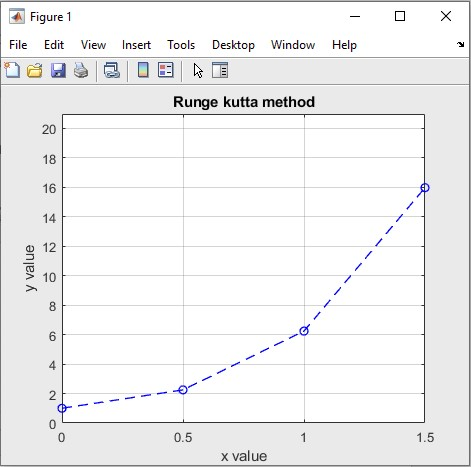Output of Runge Kutta Method in MATLAB

## Conclusion

Hopefully, you learned a little about the RK4 method and also about MATLAB programming required for solving ordinary differential equations. The Runge-Kutta method in MATLAB is a versatile and effective approach for solving ODEs. By implementing the fourth-order Runge-Kutta (RK4) method in MATLAB, you can accurately define the solutions to complex differential equations.

Throughout this blog post, we have discussed the theoretical foundation of the RK4 method, provided a practical example, and offered a MATLAB program for easy implementation. So, we can conclude that whether solving a problem analytically or by using a programming method in MATLAB, the smaller the value of h, the smaller will be the error in the calculation of the result.

Start utilizing this powerful numerical method today and unlock the potential of ODE solving in MATLAB!

Ready to take your MATLAB skills to the next level? Look no further! At MATLAB Helper, we've got you covered. From free community support to expert help and training, we've got all the resources you need to become a pro in no time. If you have any questions or queries, don't hesitate to reach out to us. Simply post a comment below or send us an email at [email protected].

And don't forget to connect with us on LinkedIn, Facebook, and Subscribe to our YouTube Channel! We're always sharing helpful tips and updates, so you can stay up-to-date on everything related to MATLAB. Plus, if you spot any bugs or errors on our website, just let us know and we'll make sure to fix it ASAP.

Ready to get started? Book your expert help with Research Assistance plan today and get personalized assistance tailored to your needs. Or, if you're looking for more comprehensive training, join one of our training modules and get hands-on experience with the latest techniques and technologies. The choice is yours – start learning and growing with MATLAB Helper today!

Education is our future. MATLAB is our feature. Happy MATLABing!

0 shares

Tags

MATLAB Programming for Differential Equations, Runge-Kutta Method, Runge-Kutta Method in MATLAB, Solving ODEs in MATLAB

## Lidar Point Cloud Data Processing

Lidar Point Cloud Data Processing

## Kalman Filter 101: State Estimation

Kalman Filter 101: State Estimation

#### MATLAB Helper ®

Use Website Chat or WhatsApp at +91-8104622179